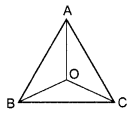# DAV Class 7 Maths Chapter 8 Worksheet 3 Solutions

The DAV Maths Book Class 7 Solutions Pdf and DAV Class 7 Maths Chapter 8 Worksheet 3 Solutions of Triangle and Its Properties offer comprehensive answers to textbook questions.

## DAV Class 7 Maths Ch 8 WS 3 Solutions

Question 1.
Which of the following can be the sides of a triangle? Measurement of sides are given in cm.
(a) 6, 4, 8
(b) 8,10, 18
(c) 3, 4,10
(d) 35, 38, 40
(a) 4 + 6 > 8, Hence triangle is possible to construct.
(b) 8 + 10 = 18, Hence triangle is not possible to construct. It is a straight line.
(c) 3 + 4 < 10, Hence triangle is not possible to construct.
(d) 35 + 38 > 40, Hence triangle is possible to construct.

Question 2.
O is a point in the interior of A ABC. Use the symbols >, < or = to make the following statements true.
(a) OA + OB __ AB
(b)OB + OC __ BC
(c)OA + OC __ AC
Now, show that AB + BC + AC < 2 (OA + OB + OC)